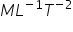(1) The body which requires greater deforming force to produce a certain change in dimension is more elastic.

Example : Ivory and steel balls are more elastic than rubber.

(2) When equal deforming force is applied on different bodies then the body which shows less deformation is more elastic.

Example : (i) For same load, more elongation is produced in rubber wire than in steel wire hence steel is more elastic than rubber.

(ii) Water is more elastic than air as volume change in water is less for same applied pressure.

(iii) Four identical balls of different materials are dropped from the same height then after collision balls rises upto different heights.

The order of their height can be given by hivory > hsteel > hrubber > hclay because Yivory > Ysteel > Yrubber > Yclay.

(3) The value of moduli of elasticity is independent of the magnitude of the stress and strain. It depends only on the nature of material of the body.

(4) For a given material there can be different moduli of elasticity depending on the type of stress applied and resulting strain.

 Name of substance Young’s modulus (Y) 1010N/m2 Bulk modulus (K) 1010N/m2 Modulus of rigidity (h) 1010N/m2 Aluminium 6.9 7.0 2.6 Brass 9.0 6.7 3.4 Copper 11.0 13.0 4.5 Iron 19.0 14.0 4.6 Steel 20.0 16.0 8.4 Tungsten 36.0 20.0 15.0 Diamond 83.0 55.0 34.0 Water – 0.22 – Glycerin – 0.45 – Air – 1.01 –

(5) The moduli of elasticity has same dimensional formula and units as that of stress since strain is dimensionless. \ Dimensional formulawhile units dyne/cm2 or Newton/m2.

(6) Greater the value of moduli of elasticity more elastic is the material. But as Y µ (1/l), K µ (1/DV) and h µ (1/f) for a constant stress, so smaller change in shape or size for a given stress corresponds to greater elasticity.

(7) The moduli of elasticity Y and h exist only for solids as liquids and gases cannot be deformed along one dimension only and also cannot sustain shear strain. However K exist for all states of matter viz. solid, liquid or gas.

(8) Gases being most compressible are least elastic while solids are most i.e. the bulk modulus of gas is very low while that for liquids and solids is very high. Ksolid > Kliquid > Kgas

(9) For a rigid body l, DV or f = 0 so Y, K or h will be ¥, i.e. elasticity of a rigid body is infinite.

Diamond and carborundum are nearest approach to rigid bodies.

(10) In a suspension bridge there is a stretch in the ropes by the load of the bridge. Due to which length of rope changes. Hence Young’s modulus of elasticity is involved.

(11) In an automobile tyre as the air is compressed, volume of the air in tyre changes, hence the bulk modulus of elasticity is involved.

(12) In transmitting power, an automobile shaft is sheared as it rotates, so shearing strain is set up, hence modulus of rigidity is involved.

(13) The shape of rubber heels changes under stress, so modulus of rigidity is involved.

Related Keywords